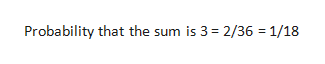2.) Two distinct fair dice are rolled at the same time and the number of dots appearing on both are counted. Find the probability that this sum is (Show your Work)A.) 3 or 12 B.) at least a 6

Question

2.) Two distinct fair dice are rolled at the same time and the number of dots appearing on both are counted. Find the probability that this sum is (Show your Work)

A.) 3 or 12 B.) at least a 6

Step 1
1. Probability that the sum is 3

It is possible only in following combinations:

• Dice one has 1 dice and dice 2 has two dots
• Dice 1 has two dots and dice 2 has one dot.

Total number of combinations that two dice can make = 6*6 =36

Step 2help_outlineImage TranscriptioncloseProbability that the sum is 3 2/36 1/18 fullscreen
Step 3

Probability that the sum is 3 = 2/36 = 1/18

Probability that the sum is 12

Case...

Want to see the full answer?

See Solution

Want to see this answer and more?

Our solutions are written by experts, many with advanced degrees, and available 24/7

See Solution
Tagged in

Other